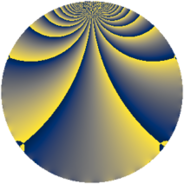# Properties

 Label 2300.2.eLevel $2300$ Weight $2$ Character orbit 2300.e Rep. character $\chi_{2300}(551,\cdot)$ Character field $\Q$ Dimension $222$ Sturm bound $720$

# Related objects

## Defining parameters

 Level: $$N$$ $$=$$ $$2300 = 2^{2} \cdot 5^{2} \cdot 23$$ Weight: $$k$$ $$=$$ $$2$$ Character orbit: $$[\chi]$$ $$=$$ 2300.e (of order $$2$$ and degree $$1$$) Character conductor: $$\operatorname{cond}(\chi)$$ $$=$$ $$92$$ Character field: $$\Q$$ Sturm bound: $$720$$

## Dimensions

The following table gives the dimensions of various subspaces of $$M_{2}(2300, [\chi])$$.

Total New Old
Modular forms 372 234 138
Cusp forms 348 222 126
Eisenstein series 24 12 12

## Trace form

 $$222q - 4q^{4} - 3q^{6} + 3q^{8} - 206q^{9} + O(q^{10})$$ $$222q - 4q^{4} - 3q^{6} + 3q^{8} - 206q^{9} - q^{12} + 4q^{13} - 12q^{16} + 13q^{18} + 16q^{24} + q^{26} + 12q^{29} - 33q^{36} - 4q^{41} - 53q^{48} + 198q^{49} - 53q^{52} - 7q^{54} + 9q^{58} + 5q^{62} - 61q^{64} + 52q^{69} + 67q^{72} + 4q^{73} + 56q^{77} - 99q^{78} + 94q^{81} - 23q^{82} - 40q^{92} + 72q^{93} - 7q^{94} - 5q^{96} - 16q^{98} + O(q^{100})$$

## Decomposition of $$S_{2}^{\mathrm{new}}(2300, [\chi])$$ into newform subspaces

The newforms in this space have not yet been added to the LMFDB.

## Decomposition of $$S_{2}^{\mathrm{old}}(2300, [\chi])$$ into lower level spaces

$$S_{2}^{\mathrm{old}}(2300, [\chi]) \cong$$ $$S_{2}^{\mathrm{new}}(92, [\chi])$$$$^{\oplus 3}$$$$\oplus$$$$S_{2}^{\mathrm{new}}(460, [\chi])$$$$^{\oplus 2}$$